# Axiom of extensionality

(diff) ← Older revision | Latest revision (diff) | Newer revision → (diff)

One of the axioms of set theory, asserting that two sets are equal if they contain the same elements: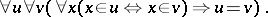In a language not containing the equality symbol and having only one predicate symbol, the axiom of extensionality has the formThe axiom of extensionality has no real importance for the formalization of mathematics in the Zermelo–Fraenkel system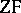. Anything that can be constructed within the systemcan be formalized in a system without the axiom of extensionality. Letbe the system obtained fromby removing the axiom of extensionality and by replacing formulas of the form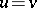in the remaining axioms by the formulaThen it can be shown that there exists an interpretation ofin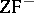. A similar assertion is valid for the theory of types.

For Quine's system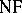, obtained from the theory of types by the "erasure" of the type indices, the situation is different: It is not possible to interpretin. The system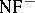(without the axiom of extensionality) is a rather weak system, and its consistency can be proved in formal arithmetic. The system, however, is not weaker than the theory of types with the axiom of infinity.

How to Cite This Entry:
Axiom of extensionality. Encyclopedia of Mathematics. URL: http://encyclopediaofmath.org/index.php?title=Axiom_of_extensionality&oldid=16856
This article was adapted from an original article by V.N. Grishin (originator), which appeared in Encyclopedia of Mathematics - ISBN 1402006098. See original article